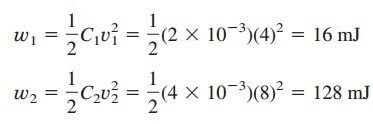# Capacitor Voltage Current Capacitance Formula – What is CapacitorCapacitor voltage current capacitance formula is very important for us to learn. This is the most basic passive element in the electric circuit.

So far we have limited our study to resistive circuits. In this post, we shall introduce two new and important passive linear circuit elements: the capacitor and the inductor. Unlike resistors, which dissipate energy, capacitors and inductors do not dissipate but store energy, which can be retrieved at a later time. F or this reason, capacitors and inductors are called storage elements.

The application of resistive circuits is quite limited. With the introduction of capacitors and inductors in this post, we will be able to analyze more important and practical circuits.

We begin by introducing capacitors and describing how to combine them in series or in parallel. Later, we do the same for inductors. As typical applications, we explore how capacitors are combined with op amps to form integrators, differentiators, and analog computers.

## Capacitor Voltage Current Capacitance Formula

A capacitor is a passive element designed to store energy in its electric field. Besides resistors, capacitors are the most common electrical components. Capacitors are used extensively in electronics, communications, computers, and power systems. For example, they are used in the tuning circuits of radio receivers and as dynamic memory elements in computer systems.

A capacitor is typically constructed as depicted in Figure.(1).

A capacitor consists of two conducting plates separated by an insulator (or dielectric).

In many practical applications, the plates may be aluminium foil while the dielectric may be air, ceramic, paper, or mica.

When a voltage source v is connected to the capacitor, as in Figure.(2), the source deposits a positive charge q on one plate and a negative charge q on the other.

The capacitor is said to store the electric charge. The amount of charge stored, represented by q, is directly proportional to the applied voltage v so that

where C, the constant of proportionality, is known as the capacitance of the capacitor. The unit of capacitance is the farad (F), in honour of the English physicist Michael Faraday (1791–1867). From Equation.(1), we may derive the following definition.

Capacitance is the ratio of the charge on one plate of a capacitor to the voltage difference between the two plates, measured in farads (F).

Note from Equation.(1) that 1 farad = 1 coulomb/volt.

Although the capacitance C of a capacitor is the ratio of the charge q per plate to the applied voltage v, it does not depend on q or v. It depends on the physical dimensions of the capacitor. For example, the parallel plate capacitor shown in Figure.(1), the capacitance is given by

where A is the surface area of each plate, d is the distance between the plates and ϵ is the permittivity of the dielectric material between the plates. Although Equation.(2) applies to only parallel-plate capacitors, we may infer from it that, in general, three factors determine the value of the capacitance:

1. The surface area of the plates—the larger the area, the greater the capacitance.
2. The spacing between the plates—the smaller the spacing, the greater the capacitance.
3. The permittivity of the material—the higher the permittivity, the greater the capacitance.

Capacitors are commercially available in different values and types. Typically, capacitors have values in the picofarad (pF) to microfarad (μF) range. They are described by the dielectric material they are made of and by whether they are of fixed or variable type. Figure.(3) shows the circuit symbols for fixed and variable capacitors.

Note that according to the passive sign convention, if v > 0 and i > 0 or if v < 0 and i < 0, the capacitor is being charged, and if v · i < 0, the capacitor is discharging.

Figure.(4) shows common types of fixed-value capacitors. Polyester capacitors are light in weight, stable, and their change with temperature is predictable. Instead of polyester, other dielectric materials such as mica and polystyrene may be used. Film capacitors are rolled and housed in metal or plastic films. Electrolytic capacitors produce very high capacitance.

Figure.(5) shows the most common types of variable capacitors. The capacitance of a trimmer (or padder) capacitor is often placed in parallel with another capacitor so that the equivalent capacitance can be varied slightly.

The capacitance of the variable air capacitor (meshed plates) is varied by turning the shaft. Variable capacitors are used in radio receivers allowing one to tune to various stations. In addition, capacitors are used to block dc, pass ac, shift phase, store energy, start motors, and suppress noise.

To obtain the current-voltage relationship of the capacitor, we take the derivative of both sides of Equation.(1). Since

differentiating both sides of Equation.(1) gives

This is the current-voltage relationship for a capacitor, assuming the passive sign convention. The relationship is illustrated in Figure.(6) for a capacitor whose capacitance is independent of voltage.

Capacitors that satisfy Equation.(4) are said to be linear. For a nonlinear capacitor, the plot of the current-voltage relationship is not a straight line. Although some capacitors are nonlinear, most are linear. We will assume linear capacitors in this post.

The voltage-current relation of the capacitor can be obtained by integrating both sides of Equation.(4). We get

or

where v(t0) = q(t0)/C is the voltage across the capacitor at time t0. Equation.(6) shows that the capacitor voltage depends on the past history of the capacitor current. Hence, the capacitor has memory—a property that is often exploited.

The instantaneous power delivered to the capacitor is

The energy stored in the capacitor is therefore

We note that v(−∞) = 0 because the capacitor was uncharged at t = −∞. Thus,

Using Equation.(1), we may rewrite Equation.(9) as

Equations.(9) or (10) represents the energy stored in the electric field that exists between the plates of the capacitor. This energy can be retrieved since an ideal capacitor cannot dissipate energy. In fact, the word capacitor is derived from this element’s capacity to store energy in an electric field.

We should note the following important properties of a capacitor:

1. Note from Equation.(4) that when the voltage across a capacitor is not changing with time (i.e., dc voltage), the current through the capacitor is zero. Thus,

A capacitor is an open circuit to dc.

However, if a battery (dc voltage) is connected across a capacitor, the capacitor charges.

2. The voltage on the capacitor must be continuous.

The voltage on a capacitor cannot change abruptly.

The capacitor resists an abrupt change in the voltage across it. According to Equation.(4), a discontinuous change in voltage requires an infinite current, which is physically impossible. For example, the voltage across a capacitor may take the form shown in Figure.(7a), whereas it is not physically possible for the capacitor voltage to take the form shown in Figure.(7b) because of the abrupt changes. Conversely, the current through a capacitor can change instantaneously.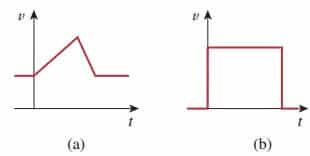Figure 7. The voltage across a capacitor: (a) allowed, (b) not allowable; an abrupt change is not possible.

3. The ideal capacitor does not dissipate energy. It takes power from the circuit when storing energy in its field and returns previously-stored energy when delivering power to the circuit.

4. A real, nonideal capacitor has a parallel-model leakage resistance, as shown in Figure.(8). The leakage resistance may be as high as 100  M Ω and can be neglected for most practical applications. For this reason, we will assume ideal capacitors in this post.

## Capacitor Voltage Current Capacitance Formula Examples

1. (a) Calculate the charge stored on a 3-pF capacitor with 20 V across it. (b) Find the energy stored in the capacitor.

Solution:
(a) Since q = Cv,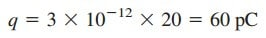(b) The energy stored is2. The voltage across a 5- μF capacitor is

v(t) = 10 cos 6000t V

Calculate the current through it.

Solution:
By definition, the current is3. Determine the voltage across a 2- μF capacitor if the current through it is
i(t) = 6e −3000t mA
Assume that the initial capacitor voltage is zero.

Solution:
Sincethen,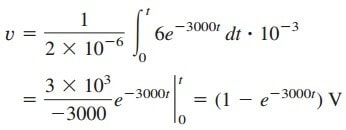4. Determine the current through a 200-μF capacitor whose voltage is

shown in Figure.(9).

Solution:
The voltage waveform can be described mathematically as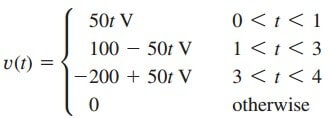Since i = C dv/dt and C = 200 μF, we take the derivative of v to obtainThus, the current waveform is as shown in Figure.(10).

5. Obtain the energy stored in each capacitor in Figure.(11a) under dc
conditions.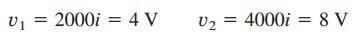and the energies stored in them are